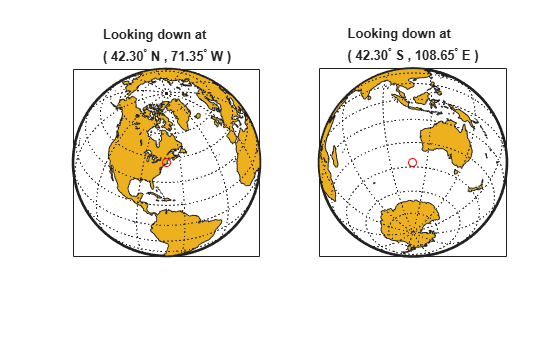# antipode

Point on opposite side of globe

## Syntax

```[newlat,newlon] = antipode(lat,lon) [newlat,newlon] = antipode(lat,lon,angleunits) ```

## Description

`[newlat,newlon] = antipode(lat,lon)` returns the geographic coordinates of the points exactly opposite on the globe from the input points given by `lat` and `lon`. All angles are in degrees.

`[newlat,newlon] = antipode(lat,lon,angleunits)` where `angleunits` specifies the input and output units as either `'degrees'` or `'radians'`. It can be abbreviated and is case-insensitive.

## Examples

### Find Antipode of Given Point

Given a point (43ºN, 15ºE), find its antipode:

```[newlat,newlong] = antipode(43,15) newlat = -43 newlong = -165 ```

or (43ºS, 165ºW).

### Find Antipode of North and South Poles

Perhaps the most obvious antipodal points are the North and South Poles. The function `antipode` demonstrates this:

```[newlat,newlong] = antipode(90,0,'degrees') newlat = -90 newlong = 180 ```

Note that in this case longitudes are irrelevant because all meridians converge at the poles.

### Find Antipode of MathWorks Headquarters

This example shows how to find the antipode of the location of the MathWorks corporate headquarters in Natick, Massachusetts. The example maps the headquarters location and its antipode in an orthographic projection.

Specify latitude and longitude as degree-minutes-seconds and then convert to decimal degrees.

`mwlat = dms2degrees([ 42 18 2.5])`
```mwlat = 42.3007 ```
`mwlon = dms2degrees([-71 21 7.9])`
```mwlon = -71.3522 ```

Find the antipode.

`[amwlat amwlon] = antipode(mwlat,mwlon)`
```amwlat = -42.3007 ```
```amwlon = 108.6478 ```

Prove that these points are antipodes. The `distance` function shows them to be 180 degrees apart.

`dist = distance(mwlat,mwlon,amwlat,amwlon)`
```dist = 180.0000 ```

Generate a map centered on the original point and then another map centered on the antipode.

```figure subplot(1,2,1) axesm ('MapProjection','ortho','origin',[mwlat mwlon],... 'frame','on','grid','on') load coastlines geoshow(coastlat,coastlon,'displaytype','polygon') geoshow(mwlat,mwlon,'Marker','o','Color','red') title(sprintf('Looking down at\n(%s,%s)', ... angl2str(mwlat,'ns'), angl2str(mwlon,'ew'))) subplot(1,2,2) axesm ('MapProjection','ortho','origin',[amwlat amwlon],... 'frame','on','grid','on') geoshow(coastlat,coastlon,'displaytype','polygon') geoshow(amwlat,amwlon,'Marker','o','Color','red') title(sprintf('Looking down at\n(%s,%s)', ... angl2str(amwlat,'ns'), angl2str(amwlon,'ew')))```Introduced before R2006a

## SupportGet trial now